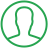# How To Import Random In Python

Last Updated : Oct 16, 2023In this article we will show you the solution of how to import random in python, consider a situation where we would like the computer to choose a random integer from a preset range, an item at random from a list, a card at random from a deck, flip a coin, and so on.

We employ the Python import random module for such reasons.

The Python import random module, as well as its various functions, programming applications, and a program to generate random numbers in Python, will be the main topics of this article.

We will now discuss the idea of how to import random numbers in python with an example.

## Step By Step Guide On How To Import Random In Python :-

### Code 1

```import random
integer1=random.randint(1,10)
integer2=random.randint(1,10)
integer3=random.randint(1,10)
integer4=random.randint(1,10)
print("The random int between 1 and 10 are:",integer1, integer2, integer3, integer4)```
1. To produce random integers, the random module is imported.
2. The variables integer1, integer2, integer3, and integer4 are declared and given random integer values between 1 and 10 using the randint() function from the random module.
3. The print() method and string concatenation are used to display the random integer numbers next to a message.
4. The message "The random integers between 1 and 10 are:" will appear after the four random integer values, which will be separated by spaces.

### Code 2

```# Python program to illustrate choice() method
import random
# prints a random value from the list
list1 = [8, 5, 6, 2, 0, 4]
print('Random number from list:', random.choice(list1))
# prints a random item from the string
string = '9674271903'
print('Random number from string:', random.choice(string))```
1. In order to use the choice() function of the random module, we import it first.
2. A list list1 with a collection of numbers is defined.
3. We choose a random element from list1 using the choice() function, then we print it. The list is sent to the function as an input to accomplish this.
4. We construct a string made up of a string of numbers.
5. Once more, we choose a character at random from a string using the choose() function, and we output it. This is accomplished by giving the function the string as an argument.

## Conclusion :-

As a result, we have successfully learned how to import random numbers in python with an example.

In this article, we've covered a variety of Python's random module functions. We have also covered a code example that simulates coin flips by using the choose() function.

I hope this article on how to import random in python helps you and the steps and method mentioned above are easy to follow and implement.

##About Pragati

Experienced coding content writer who enjoys breaking down complex concepts in programming languages like Java, Python, C, and C++. Over three years of experience producing interesting and relevant content for a variety of entities. Committed to providing concise and easy-to-understand articles that assist readers in easily understanding technology and industry trends in the world of coding and software development.